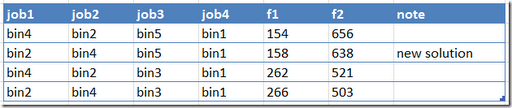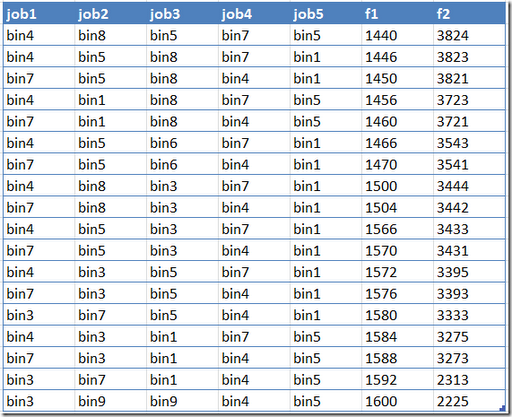## Monday, September 24, 2012

### Bi-criteria optimization

In the following paper http://nvlpubs.nist.gov/nistpubs/jres/111/2/V111.N02.A05.pdf a two objective model is developed. The usual way I see such models implemented is by adding weights in some linear fashion, e.g.:Here a somewhat unusual form is used: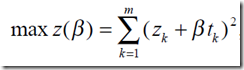Indeed when β=0 we get a single objective scheduling problem:Here the slack zk in bin k is maximized using a quadratic objective. This is not a trivial problem to solve in itself as the problem is non-convex and we need a global solver. The authors use Lingo but other suitable solvers are Baron and GloMiQo. (Looking at the data – all integer – it should not be too difficult to linearize this at the expense of extra binary variables).

When we look at the 2-objective problem formulation we can observe this can be written as:When β is fixed the last term is constant, so we are left with: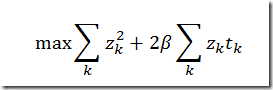Note that the paper want to maximize the slacks zk, but minimize the start time xi,ktk. I did not immediately understand how this objective can actually work. The more direct approach I would use is:or may be: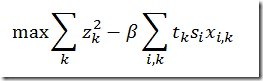Actually what is proposed in the paper is quite clever. Observe that minimizing the tksixi,k's is equivalent to maximizing tkzk (as the z is a slack indicating unused capacity).

The paper indicates it finds three different solutions. They probably use a scheme trying beta=0,0.01,0.02,.., or may be some bisection. There is a much better approach where we solve a single problem for each non-dominated point (plus one problem to detect there are no more such points). Using the separable formulation with: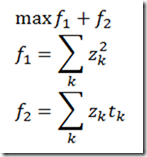we can add some equations that allow us to trace the efficient frontier. This actually gives us 4 non-dominated solutions: